# I- Purely Inductive Load1. Sketch a three-phase 100VAC RMS phase-voltage, Wye (Y) Connected power supply with aWye (Y) Connected load with purely inductive j3002 per phase.2. Assume the phase-voltage of phase-A at the source side has an angle of zero. Calculate bothmagnitudes and phase-angles for phase voltage, line voltage, phase current, and line currentat the load side. Also, compute load real power, apparent Power, and power factor.Ve V VLine_V l A ILine.V.A. P.F.(lagging or leading)

Question
1 views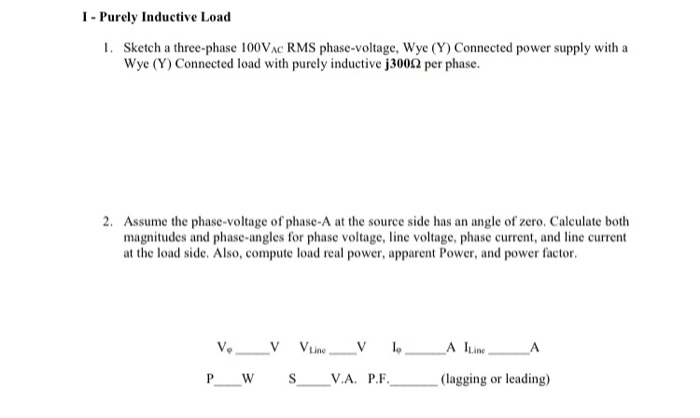help_outlineImage TranscriptioncloseI- Purely Inductive Load 1. Sketch a three-phase 100VAC RMS phase-voltage, Wye (Y) Connected power supply with a Wye (Y) Connected load with purely inductive j3002 per phase. 2. Assume the phase-voltage of phase-A at the source side has an angle of zero. Calculate both magnitudes and phase-angles for phase voltage, line voltage, phase current, and line current at the load side. Also, compute load real power, apparent Power, and power factor. Ve V VLine_V l A ILine. V.A. P.F. (lagging or leading) fullscreen
check_circle

Examination of the problem.

We are authorized to answer one question at a time since you have not mentioned which question you are looking for, so we are answering the first one, please repost your other question separately if you want to get answered.

In the given problem the supply is star connected and the load is also star connected. The R.M.S value of the supply voltage is given also the load is inductive in nature. The approach,  to solve this problem will be to first draw the sketch of the supply end after that we will draw the load end sketch. After that, both will be combined together.

Supply end

Three-phase Y at the supply end. The phase difference among each phase will be 120degrees and the value of the R.M.S voltage is given.

The phase voltages are as follows: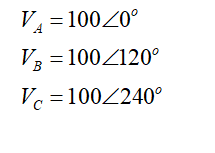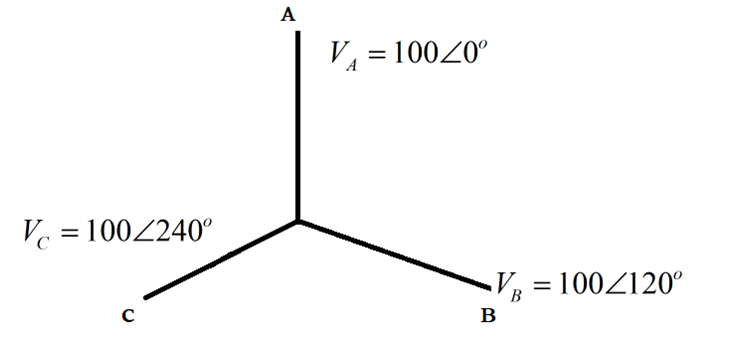The loads are purely inductive therefore the voltage will have the leading phase of 90 degrees as compared to current in the star-connected load winding.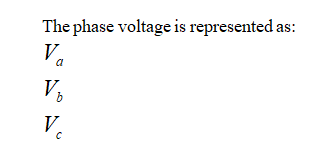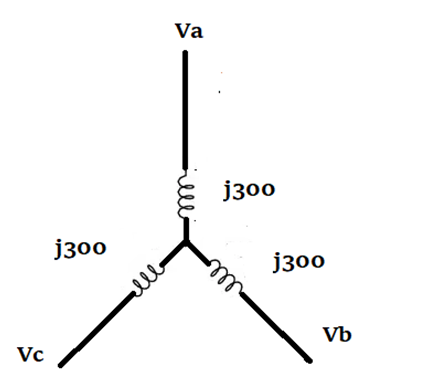...

### Want to see the full answer?

See Solution

#### Want to see this answer and more?

Solutions are written by subject experts who are available 24/7. Questions are typically answered within 1 hour.*

See Solution
*Response times may vary by subject and question.
Tagged in